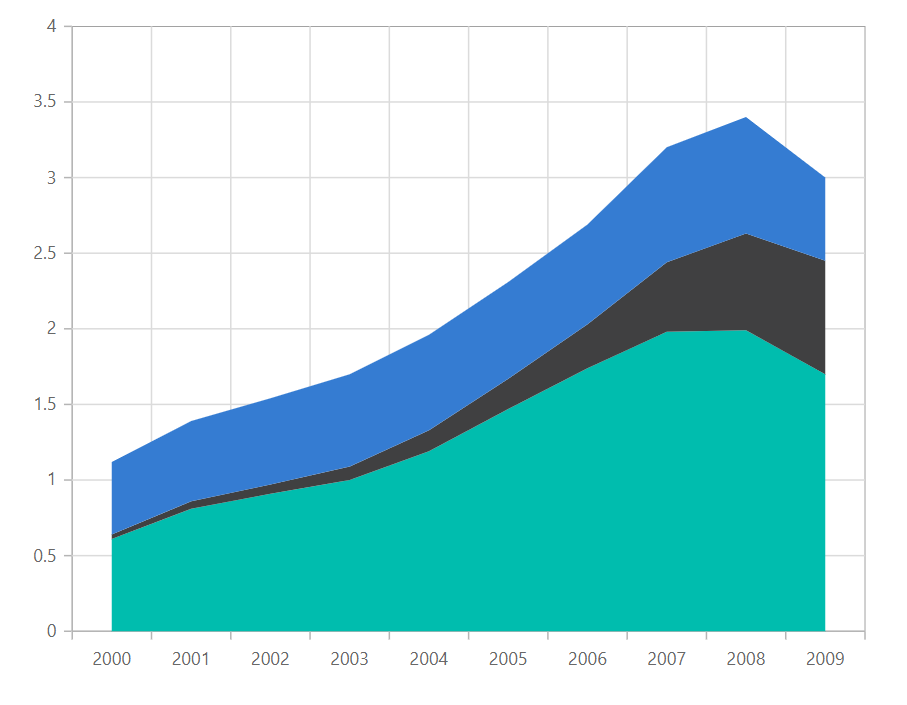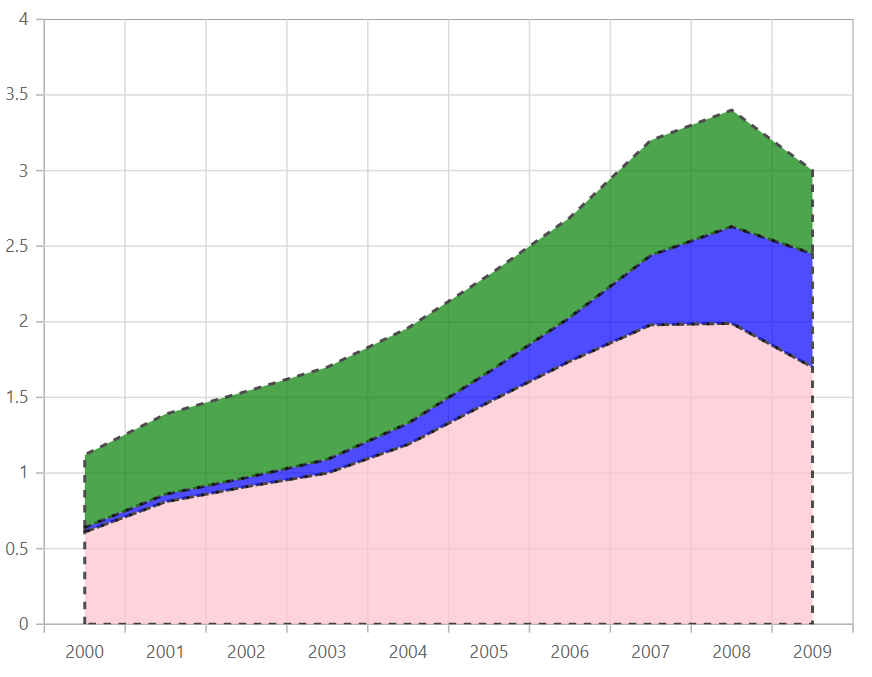# Stacked Area in Blazor Charts Component

14 Feb 20225 minutes to read

## Stacked Area

Blazor Stacked Area Chart is a chart with Y values stacked over one another in the series order. It shows the relation between individual values to the total sum of the points. To render a Stacked Area series, set the series Type as StackingArea.

``````@using Syncfusion.Blazor.Charts

<SfChart>
<ChartPrimaryXAxis ValueType="Syncfusion.Blazor.Charts.ValueType.Category"/>

<ChartSeriesCollection>
<ChartSeries DataSource="@MedalDetails" XName="X" YName="Y" Type="ChartSeriesType.StackingArea"/>
<ChartSeries DataSource="@MedalDetails" XName="X" YName="Y1" Type="ChartSeriesType.StackingArea"/>
<ChartSeries DataSource="@MedalDetails" XName="X" YName="Y2" Type="ChartSeriesType.StackingArea"/>
</ChartSeriesCollection>
</SfChart>

@code{
public class ChartData
{
public double X { get; set; }
public double Y { get; set; }
public double Y1 { get; set; }
public double Y2 { get; set; }
}

public List<ChartData> MedalDetails = new List<ChartData>
{
new ChartData{ X=2000, Y= 0.61, Y1= 0.03, Y2= 0.48},
new ChartData{ X=2001, Y= 0.81, Y1= 0.05, Y2= 0.53 },
new ChartData{ X=2002, Y= 0.91, Y1= 0.06, Y2= 0.57 },
new ChartData{ X=2003, Y= 1, Y1= 0.09, Y2= 0.61 },
new ChartData{ X=2004, Y= 1.19, Y1= 0.14, Y2= 0.63 },
new ChartData{ X=2005, Y= 1.47, Y1= 0.20, Y2= 0.64 },
new ChartData{ X=2006, Y= 1.74, Y1= 0.29, Y2= 0.66 },
new ChartData{ X=2007, Y= 1.98, Y1= 0.46, Y2= 0.76 },
new ChartData{ X=2008, Y= 1.99, Y1= 0.64, Y2= 0.77 },
new ChartData{ X=2009, Y= 1.70, Y1= 0.75, Y2= 0.55 }
};
}``````Refer to our Blazor Stacked Area Chart feature tour page to know about its other groundbreaking feature representations. Explore our Blazor Stacked Area Chart Example to know how to render and configure the Stacked Area type chart.

## Series customization

The following properties can be used to customize the Stacked Area series.

``````@using Syncfusion.Blazor.Charts

<SfChart>
<ChartPrimaryXAxis ValueType="Syncfusion.Blazor.Charts.ValueType.Category" />

<ChartSeriesCollection>
<ChartSeries DataSource="@MedalDetails" XName="X" YName="Y" Fill="pink" Opacity="0.7" DashArray="5,5" Type="ChartSeriesType.StackingArea">
<ChartSeriesBorder Width="2" Color="black"></ChartSeriesBorder>
</ChartSeries>
<ChartSeries DataSource="@MedalDetails" XName="X" YName="Y1" Fill="blue" Opacity="0.7" DashArray="5,5" Type="ChartSeriesType.StackingArea">
<ChartSeriesBorder Width="2" Color="black"></ChartSeriesBorder>
</ChartSeries>
<ChartSeries DataSource="@MedalDetails" XName="X" YName="Y2" Fill="green" Opacity="0.7" DashArray="5,5" Type="ChartSeriesType.StackingArea">
<ChartSeriesBorder Width="2" Color="black"></ChartSeriesBorder>
</ChartSeries>
</ChartSeriesCollection>
</SfChart>

@code{
public class ChartData
{
public double X { get; set; }
public double Y { get; set; }
public double Y1 { get; set; }
public double Y2 { get; set; }
}

public List<ChartData> MedalDetails = new List<ChartData>
{
new ChartData{ X=2000, Y= 0.61, Y1= 0.03, Y2= 0.48},
new ChartData{ X=2001, Y= 0.81, Y1= 0.05, Y2= 0.53 },
new ChartData{ X=2002, Y= 0.91, Y1= 0.06, Y2= 0.57 },
new ChartData{ X=2003, Y= 1, Y1= 0.09, Y2= 0.61 },
new ChartData{ X=2004, Y= 1.19, Y1= 0.14, Y2= 0.63 },
new ChartData{ X=2005, Y= 1.47, Y1= 0.20, Y2= 0.64 },
new ChartData{ X=2006, Y= 1.74, Y1= 0.29, Y2= 0.66 },
new ChartData{ X=2007, Y= 1.98, Y1= 0.46, Y2= 0.76 },
new ChartData{ X=2008, Y= 1.99, Y1= 0.64, Y2= 0.77 },
new ChartData{ X=2009, Y= 1.70, Y1= 0.75, Y2= 0.55 }
};
}``````Refer to our Blazor Charts feature tour page for its groundbreaking feature representations and also explore our Blazor Chart Example to know various chart types and how to represent time-dependent data, showing trends at equal intervals.